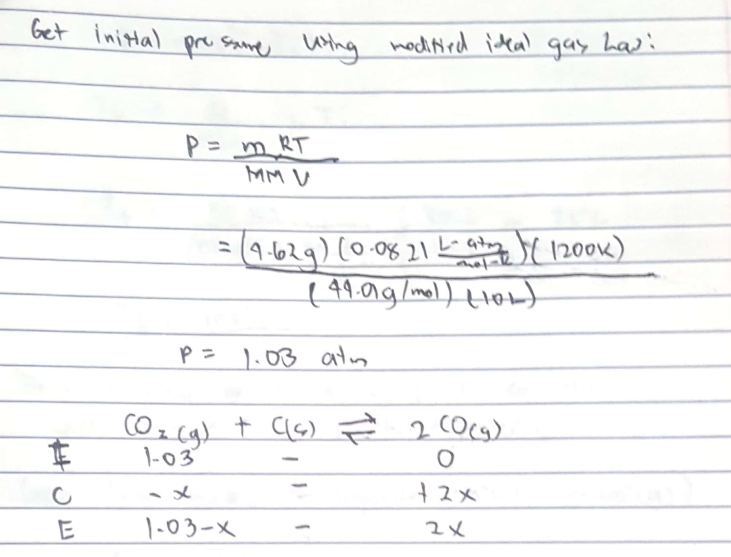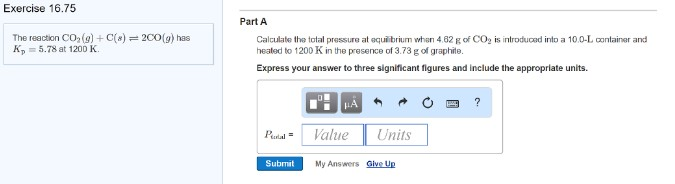# Problem: The reaction CO2 (g) + C (s) ⇌ 2CO (g) has Kp = 5.78 at 1200 K. Part ACalculate the total pressure at equilibrium when 4.62 g of CO2 is introduced into a 10.0-L container and heated to 1200 K in the presence of 3.73 g of graphite. Express your answer to three significant figures and include the appropriate units.

###### FREE Expert Solution95% (185 ratings)###### Problem Details

The reaction CO2 (g) + C (s) ⇌ 2CO (g) has Kp = 5.78 at 1200 K.

Part A

Calculate the total pressure at equilibrium when 4.62 g of CO2 is introduced into a 10.0-L container and heated to 1200 K in the presence of 3.73 g of graphite. Express your answer to three significant figures and include the appropriate units.